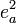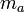# Significance Tests on Trend Plots$e_{a+b}^2=\frac{[n_a (n_a-1) e_a^2 +n_b (n_b-1) e_b^2+(n_a n_b)/(n_a+n_b ) (m_a-m_b )^2 ]}{ (n_a+n_b )(n_a+n_b-1)}$
where$n_a$ is the effective sample size of the first group,$e_a^2$ is the squared standard error of the first group,$m_a$ is the relevant statistic (proportion, mean or coefficient, depending upon the data) of the first group and the second group’s terms are denoted with the subscript b (see: Baker, R. W. R. and J. A. Nissim (1963), "Expressions for Combining Standard Errors of Two Groups and for Sequential Standard Error," Nature, 198 (8 June 1963).